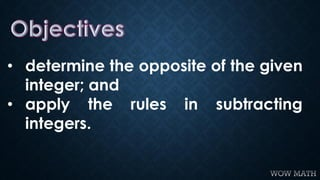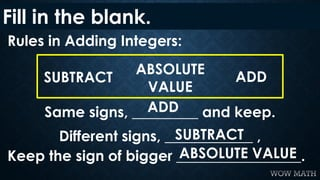Diese Präsentation wurde erfolgreich gemeldet.

# Subtraction of Integers

1

Teilen×
1 von 10
1 von 10

# Subtraction of Integers

1

Teilen

For more instructional resources, CLICK me here and DON'T FORGET TO SUBSCRIBE! 
https://tinyurl.com/y9muob6q

LIKE and FOLLOW me here! 
https://tinyurl.com/ycjp8r7u
https://tinyurl.com/ybo27k2u

For more instructional resources, CLICK me here and DON'T FORGET TO SUBSCRIBE! 
https://tinyurl.com/y9muob6q

LIKE and FOLLOW me here! 
https://tinyurl.com/ycjp8r7u
https://tinyurl.com/ybo27k2u

Reference:
Nivera, G. C. (2013), Grade 7 Mathematics: Pattern and Practicalities. Don Bosco Press Inc. Makati City, Philippines.

For more instructional resources, CLICK me here and DON'T FORGET TO SUBSCRIBE! 
https://tinyurl.com/y9muob6q

LIKE and FOLLOW me here! 
https://tinyurl.com/ycjp8r7u
https://tinyurl.com/ybo27k2u

For more instructional resources, CLICK me here and DON'T FORGET TO SUBSCRIBE! 
https://tinyurl.com/y9muob6q

LIKE and FOLLOW me here! 
https://tinyurl.com/ycjp8r7u
https://tinyurl.com/ybo27k2u

Reference:
Nivera, G. C. (2013), Grade 7 Mathematics: Pattern and Practicalities. Don Bosco Press Inc. Makati City, Philippines.

## Weitere Verwandte Inhalte

### Ähnliche Bücher

Kostenlos mit einer 14-tägigen Testversion von Scribd

Alle anzeigen

### Ähnliche Hörbücher

Kostenlos mit einer 14-tägigen Testversion von Scribd

Alle anzeigen

### Subtraction of Integers

1. 1. Grade 7 – Mathematics Quarter I SUBTRACTION OF INTEGERS
2. 2. • determine the opposite of the given integer; and • apply the rules in subtracting integers.
3. 3. Rules in Adding Integers: Fill in the blank. Same signs, _________ and keep. Different signs, ____________ , Keep the sign of bigger _________________. ADDSUBTRACT ABSOLUTE VALUE ADD SUBTRACT ABSOLUTE VALUE
4. 4. “What’s the OPPOSITE?” -10 14 -9 7 31. -3 = 2. 10 = 3. -14 = 4. 9 = 5. -7 =
5. 5. SUBTRACTION OF INTEGERS “adding the opposites” a - b a - (-b) a KEEP – CHANGE - CHANGE + (-b) KEEP CHANGE CHANGE a + b KEEP CHANGE CHANGE - proceed to ADDITION rule
6. 6. SUBTRACTION OF INTEGERS “adding the opposites” KEEP – CHANGE - CHANGE GIVEN KEEP CHANGE (adding) CHANGE (opposite) ANSWER 8 - 11 -12 – (-16) 10 – (- 6) -14 - 8 8 + (-11) 8 + (-11) = -3 -12 + 16 -12 + 16 = 4 10 + 6 10 + 6 = 16 -14 + (-8) -14 + (-8)= -22
7. 7. SUBTRACTION OF INTEGERS 2. -7 - 10 = 1. 3 - (-5) = = 83 + 5 = -17-7 + (-10) 3. -40 - 20 = = -60-40 + (-20) 4. 15 - 9 = = 615 + (-9) 5. 10 – 9 – (-4) = = 14 10 +(-9)+ 4 +(-9) = 5
8. 8. SUBTRACTION OF INTEGERS -15 - ______ = 10 = -15 + (-10)-15 - 10 1. What number must be subtracted from -15 to get 10? = -25 (-25) 8 - ______ = -4 = 8 + 48 - (-4) 2. What number must be subtracted from 8 to get -4? = 12 12
9. 9. Test Yourself. 2. -24 - 9 = 1. 2 - (-5) = 4. 3 - (-6) - 8 = 3. -12 - (-18) = 5. 9 - (-1) - (-3) =
10. 10. Answer Key 1. 7 2. -33 3. 6 4. 1 5. 13